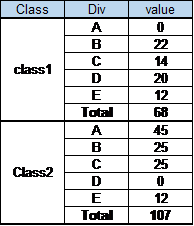# New to QlikView

Discussion board where members can get started with QlikView.

Announcements
Modernize Your QlikView Deployment webinar, Nov. 3rd. REGISTER
cancel
Showing results for
Did you mean:
HighlightedNot applicable

## compare values with Partial Sum

Hi People,

I have an pivot table like below. And I need to compare each and every value in the value column for the specific class and calculate percentage.I need the output like below.  The Percentage column is calculated by comparing the values in the value column with corresponding partial sums. I am pretty new to Qlikview. Kindly help me.

Class Div are Dimensions and value is Expression1 Solution

Accepted Solutions
HighlightedSpecialist II

Try this

Sum(Value) / Sum(Total <Class> Value)

2 Replies
HighlightedSpecialist II

Try this

Sum(Value) / Sum(Total <Class> Value)

HighlightedMVP

you can use the Total to calculate the value at the Class level

sum(value)

/

sum(Total <Class> value)